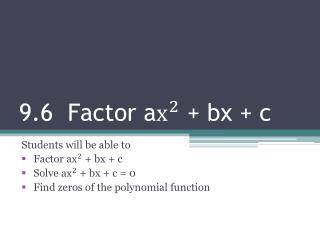DownloadDownload Presentation9.6 Factor a + bx + c

# 9.6 Factor a + bx + c

Télécharger la présentation## 9.6 Factor a + bx + c

- - - - - - - - - - - - - - - - - - - - - - - - - - - E N D - - - - - - - - - - - - - - - - - - - - - - - - - - -
##### Presentation Transcript

1. 9.6 Factor a + bx + c Students will be able to Factor a + bx + c Solve a + bx + c = 0 Find zeros of the polynomial function

2. Factor by grouping • Find a GCF in the first two terms. • Find a GCF in the last two terms. • Find common factors. 5x + 15 2x(x + 3) + 5(x + 3) (x + 3)(2x + 5)

3. Factor: 12 - 9x + 8x - 6

4. Factor: 20 - 5x - 8x + 2

5. a + bx +c Now! Factor 2 + 11x + 12 ac24 3 2x(x + 4) + 3(x + 4) 8 11 b (x + 4)(2x + 3)

6. Factor: 3 - 10x - 8

7. Factor: 15- 16x + 4

8. Solve. -3 - 13x = 4 2 - 13x + 6 = 0

9. A rectangle’s length is 5 feet more than 4 times its width. The area is 6 square feet. What is the width?

10. A baseball is hit into the air from a height of 2 feet at an initial velocity of 31 feet per second. For how many seconds is it in the air?

11. A soccer goalie throws a ball into the air at an initial height of 8 feet and an initial velocity of 28 feet per second. How long will it take to hit the ground?

12. Computer Practice • http://www.ltcconline.net/greenl/java/BasicAlgebra/AC/AC.html • 2. ttp://strader.cehd.tamu.edu/Mathematics/Algebra/AlgebraTiles/AlgebraTiles2.html